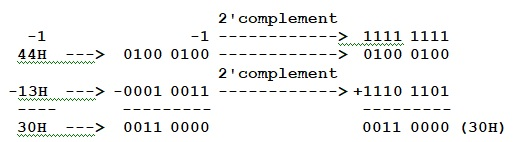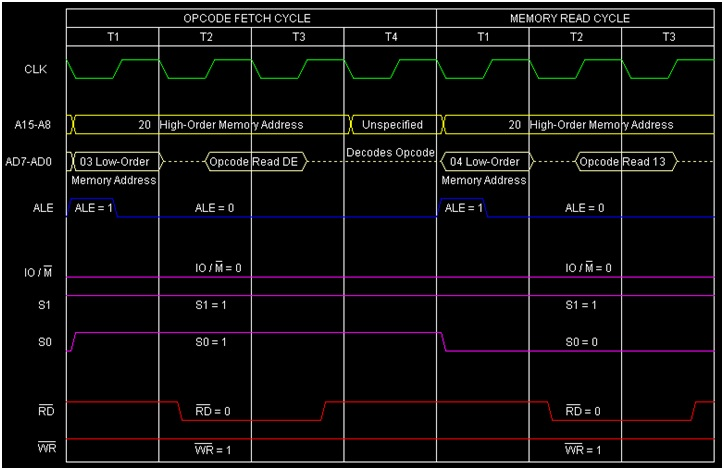# Instruction type SBI d8 in 8085 Microprocessor

In 8085 Instruction set, SBI is a mnemonic that stands for “Subtract with Borrow Immediate from Accumulator” and here d8 stands for any 8-bit data as operand. This instruction is used to subtract 8-bit immediate data from the Accumulator along with the carry (borrow) value. The result of subtraction will be stored in the Accumulator. As this is an arithmetic instruction, so the flags are affected based on the result produced. It occupies 2 consecutive Bytes in memory.

Mnemonics, Operand Opcode(in HEX) Bytes
SBI Data DE 2

Let us consider SBI 13H as a sample instruction falling in this category. It is a 2-Byte instruction. Let us consider the initial content of Accumulator is 44H. The Cy bit has been set. So 13H will get deducted from Accumulator’s content 44H along with carry bit. The internal calculations have been shown belowThe result of execution of this instruction has been depicted in the following tracing table −

Before After

(A)

44H 30H

(F)

Cy=1, other flag bits=any value Cy=0, AC=1,S=0,P=1,Z=0

Cy=0 will indicate that the result is positive.